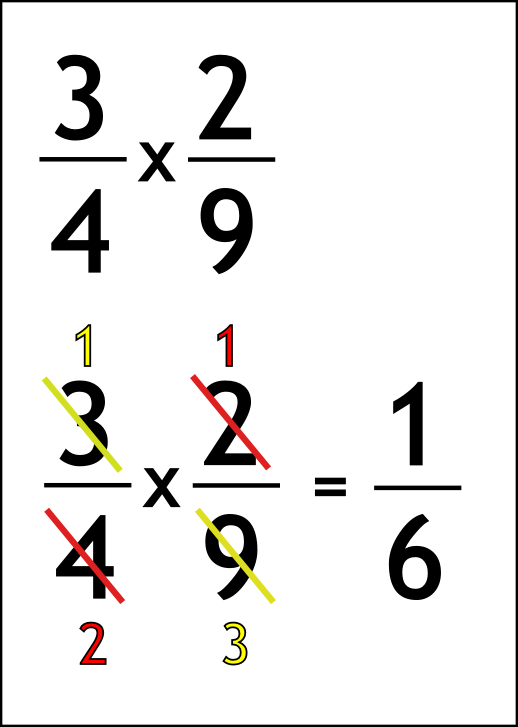The Singing Hedgehog Guide to:
Multiplying Fractions 1

When multiplying fractions we have to remember:

IT'S A CROSS SO CROSS THINGS OUT!

We just simplify the question then multiply across:Write out the question

Look for common factors (numbers to divide by)

3 and 9 are both divisible by 3 to leave 1 and 3

4 and 2 are both divisible by 2 to leave 2 and 1

Finally just multiply across

next page

fractions index